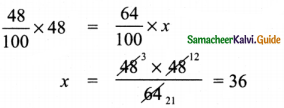Tamilnadu State Board New Syllabus Samacheer Kalvi 8th Maths Guide Pdf Chapter 4 Life Mathematics Ex 4.1 Text Book Back Questions and Answers, Notes.

## Tamilnadu Samacheer Kalvi 8th Maths Solutions Chapter 4 Life Mathematics Ex 4.1

Question 1.
Fill in the blanks:
(i) If 30 % of x is 150, then x is _______ .
500
Hint:
Given 30% of x is 150
i.e $$\frac{30}{100}$$ × x = 150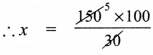∴ x = 500(ii) 2 minutes is _______ % to an hour.
3$$\frac{1}{3}$$%
Hint:
Let 2 min be x% of an hour
and 1 hr = 60mm
x% = $$\frac{2}{60} \times 100=\frac{200}{60}=\frac{10}{3}=3 \frac{1}{3}$$
x = 3$$\frac{1}{3}$$%

(iii) If x% of x = 25, then x = _______ .
50
Hint:
Given that x% of x is 25
∴ $$\frac{x}{100}$$ × x = 25
∴ x2 = 25 × 100 = 2500
∴ x = √2500 = 50

(iv) In a school of 1400 students, there are 420 girls. The percentage of boys in the school is _______ .
70
Hint:
Given total number of students in school = 1400
Number of girls in school = 420
∴ Number of boys in school = 1400 – 420 = 980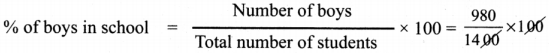= $$\frac{980}{14}$$ = 70
% of boys = 70%(v) 0.5252 is _______ %.
52.52%
Hint:
Given a number, and to express as a percentage, we need to multiply by 100
∴ to express 0.5252 as percentage, we should multiply by 100
∴ 0.5252 × 100 = 52.52%

Question 2.
Rewrite each underlined part using percentage language.
(i) One half of the cake is distributed to the children.
50% of the cake is distributed to the children
Hint:
One half is nothing but $$\frac { 1 }{ 2 }$$
as percentage, we need to multiply by 100
∴ $$\frac { 1 }{ 2 }$$ × 100 = 50%

(ii) Aparna scored 7.5 points out of 10 in a competition.
Aparna scored 75% in a competition
Hint:
7.5 points out of 10 is $$\frac{7.5}{10}$$ = 0.75
For percentage, we need to multiply by 100
We get 0.75 × 100 = 75%

(iii) The statue was made of pure silver.
The statue was made of 100% pure silver
Hint:
Pure silver means there are no other metals
so, 100 out of 100 parts is made of silver = $$\frac{100}{100}$$
∴ to express as percentage, $$\frac{100}{100}$$ × 100% = 100%(iv) 48 out of 50 students participated in sports.
96% students participated in sports.
Hint:
48 out of 50 students in fraction form is $$\frac{48}{50}$$
As a percentage, we need to multiply by 100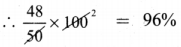(v) Only 2 persons out of 3 will be selected in the interview.
Only 66$$\frac{2}{3}$$% will be selected in the interview.
Hint:
2 out of 3 in fraction form is $$\frac{2}{3}$$
to express as percentage, we need to multiply by 100
$$\frac{2}{3} \times 100=\frac{200}{3}=66 \frac{2}{3} \%$$

Question 3.
48 is 32% of which number?
Let the number required to be found be ‘x’
Given that 32% of x is 48
i.e., $$\frac{32}{100}$$ × x = 48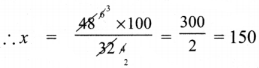∴ x = 150

Question 4.
What is 25% of 30% of 400?
Required to find 25% of 30% of 400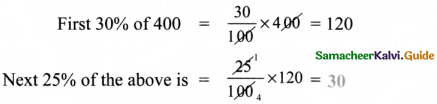Question 5.
If a car is sold for ₹ 2,00,000 from its original price of ₹ 3,00,000, then find the percentage of decrease in the value of the car.original price of car = ₹ 3,00,000
actual selling price of car = ₹ 2,00,000
Decrease in amount from original = 3,00,000 – 2,00,000 = 1,00,000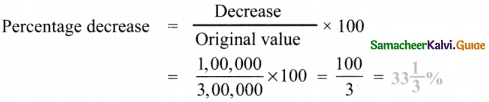Question 6.
If the difference between 75% of a number and 60% of the same number is 82.5, then find 20% of that number.
Given that 75% of number less 60% of number is 82.5
Let the number be ‘x’
∴ $$\frac{75}{100}$$ x x – $$\frac{60}{100}$$ x x = 82.5
∴ 0.75 x – 0.60 x = 82.5
∴ 0.15 x = 82.5
∴ x = $$\frac{82.5}{0.15}=\frac{8250}{15}$$ = 550
Required to find 20% of number ie 20% of x.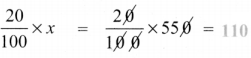Question 7.
A number when increased by 18% gives 236. Find the number.
Let the number be x. Given that when it is increased by 18%, we get 236.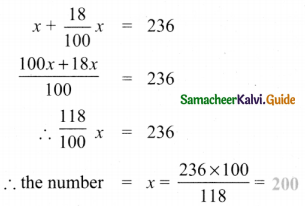Question 8.
A number when decreased by 20% gives 80. Find the number.
Let the number be x. Given that when it is increased by 20% we get 80.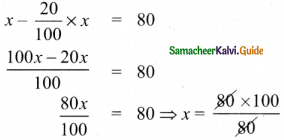x = 100Question 9.
A number is increased by 25% and then decreased by 20%. Find the percentage change in that number.
Method 1.
Let the number be x.
First it is increased by 25%
∴ It becomes x + $$\frac{25}{100}$$ × x = $$\frac{125}{100}$$
Secondary it is decreased by 20%
$$\frac{125 x}{100}-\frac{20}{100} \times \frac{125}{100} x=\frac{125}{100} x \times \frac{80}{100}=x$$
Now we get back x, therefore there is no change.
Hence percentage change in that number is 0%

Method 2.
[to understand, let us assume that number is 100]
So, first when we increase by 25%, we get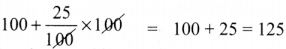Now this 125 is decreased by 20%, we get
125 – $$\frac{25}{100}$$ × 125 = 125 – 25 = 100
∴ We get back 100 ⇒ No change
Hence percentage change in that number is 0%Question 10.
The ratio of boys and girls in a class is 5:3. If 16% of boys and 8% of girls failed in an examination, then find the percentage of passed students.
Let number of boys be ‘b’ and number of girls be ‘g’
Ratio of boys and girls is given as 5:3
b:g = 5:3 ⇒ $$\frac{b}{g}=\frac{5}{3}$$ …… (A)
Failure in boys = 16% = $$\frac{16}{100}$$ × b = $$\frac{16b}{100}$$
Failure in girls = 8% = $$\frac{8}{100}$$ × g = $$\frac{8g}{100}$$
Pass in boys = 100 – 16% = 84% = $$\frac{84}{100} b$$ …… (1)
Pass in girls = 100 – 8% = 92% = $$\frac{92}{100} g$$ ……. (2)
From A, we have $$\frac{b}{g}=\frac{5}{3}$$ , adding I on both sides, we get
$$\frac{b}{g}$$ + 1 = $$\frac{5}{3}$$ + 1
$$\frac{b+g}{g}=\frac{5+3}{3}=\frac{8}{3}$$
∴ g = $$\frac{3}{8}$$(b + g) ……. (3)
Similarly b = $$\frac{5}{8}$$ (b + g) ……. (4)
Total pass = pass in girls + pass in boys
= (1) + (2) = $$\frac{84}{100} b+\frac{92}{100} g$$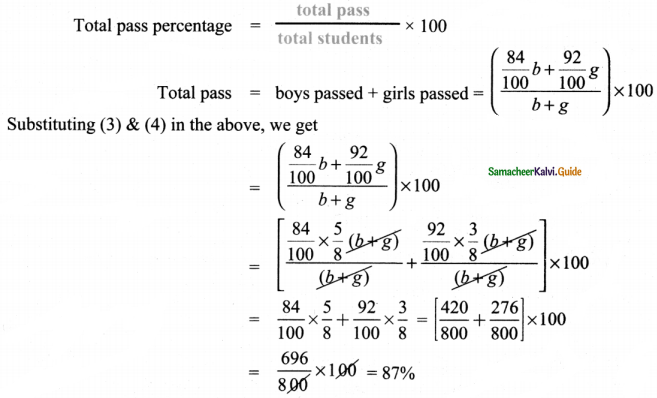Objective Type Questions

Question 11.
12% of 250 litre is the same as ________ of 150 litre.
(A) 10%
(B) 15%
(C) 20%
(D) 30%
(C) 20%
Hint:
12% of 250 = $$\frac{12}{100}$$ × 250 = 30 lit.
Percentage: $$\frac{30}{150}$$ × 100 = 20%

Question 12.
If three candidates A, B and C in a school election got 153,245 and 102 votes respectively, then the percentage of votes got by the winner is ________ .
(A) 48%
(B) 49%
(C) 50%
(D) 45%
(B) 49%
Hint:
Candidate 1: 153
Candidate 2: 245 – winner [as maximum votes)
Candidate 3: 102
Total votes = 1 + 2 + 3 = 153 + 245 + 102 = 500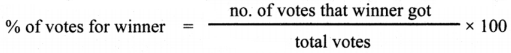= $$\frac{245}{500}$$ × 100 = 49%Question 13.
15% of 25% of 10000 = ________ .
(A) 375
(B) 400
(C) 425
(D) 475
(A) 375
Hint:
15% of 25% of 10000 is
First let us do 25% of 10,000, which is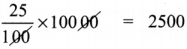Next 15% of the above is $$\frac{15}{100}$$ × 2500 = 375

Question 14.
When 60 is subtracted from 60% of a number to give 60, the number is
(A) 60
(B) 100
(C) 150
(D) 200
(D) 200
Hint:
Let the number be ‘X’
60% of the number is $$\frac{60}{100}$$ × x = $$\frac{60x}{100}$$
Given that when 60 is subtracted from 60%, we get 60
i.e $$\frac{60}{100}$$ x – 60 = 60
∴ $$\frac{60}{100}$$ x 60 + 60 = 120
∴ x = $$\frac{120 \times 100}{60}$$ = 200Question 15.
If 48% of 48 = 64% of x, then x =
(A) 64
(B) 56
(C) 42
(D) 36